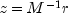JMSLTM Numerical Library 7.2.0
com.imsl.math

• All Superinterfaces:
Enclosing class:

```public static interface ConjugateGradient.Preconditioner
Public interface for the user supplied function to `ConjugateGradient` used for preconditioning.
• ### Method Summary

Methods
Modifier and Type Method and Description
`void` ```preconditioner(double[] r, double[] z)```
Used to computewhere M is the preconditioning matrix and r and z are arrays of length `n`, the order of matrix M.
• ### Methods inherited from interface com.imsl.math.ConjugateGradient.Function

`amultp`
• ### Method Detail

• #### preconditioner

```void preconditioner(double[] r,
double[] z)```
Used to computewhere M is the preconditioning matrix and r and z are arrays of length `n`, the order of matrix M.
Parameters:
`r` - an input `double` array of length `n` generated during the implementation of the `solve` method.
`z` - an output `double` array of length `n`.
JMSLTM Numerical Library 7.2.0